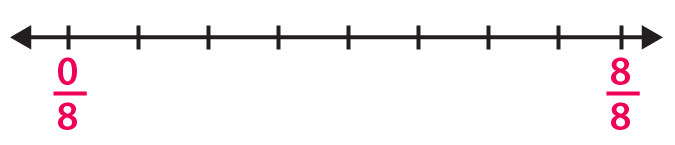# RD Sharma Solutions for Class 6 Maths Chapter 6: Fractions Exercise 6.2

RD Sharma Solutions for Class 6 are prepared with the aim of helping students ace the exam. The concepts are explained in a simple language matching the understanding capacity of the students. Exercise 6.2 of Chapter 6 mainly contains problems which are based on the methods of representing the given set of Fractions on a number line. The solutions are well structured according to the latest guidelines of the CBSE board. With the help of solutions PDF, students can solve the exercise wise problems more efficiently. RD Sharma Solutions for Class 6 Maths Chapter 6 Fractions Exercise 6.2 are provided here.

## RD Sharma Solutions for Class 6 Maths Chapter 6: Fractions Exercise 6.2 Download PDF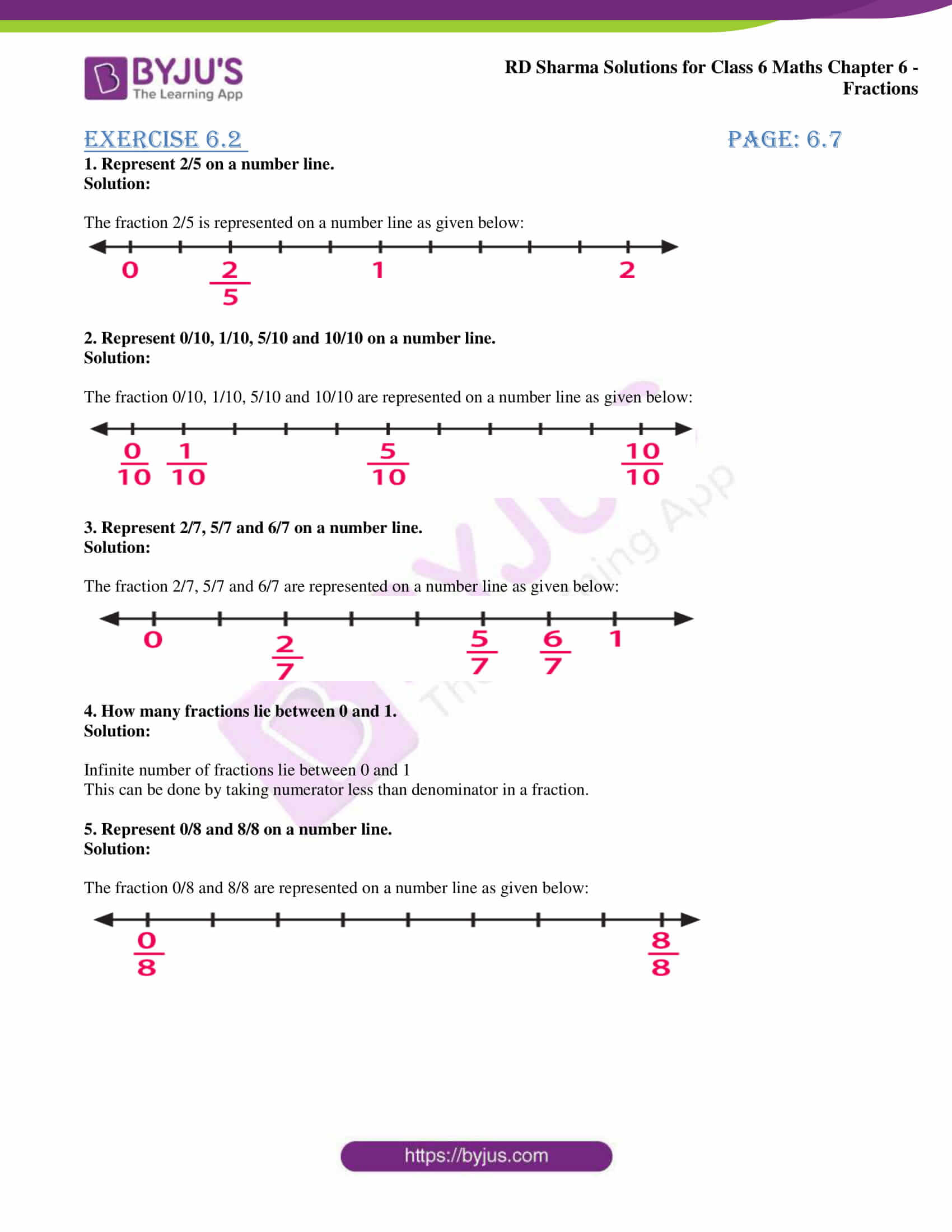### Access answers to Maths RD Sharma Solutions for Class 6 Chapter 6: Fractions Exercise 6.2

1. Represent 2/5 on a number line.

Solution:

The fraction 2/5 is represented on a number line as given below: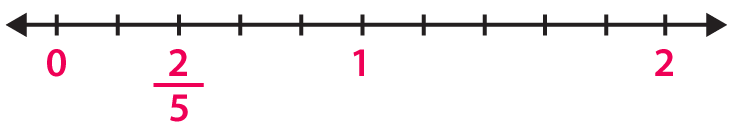2. Represent 0/10, 1/10, 5/10 and 10/10 on a number line.

Solution:

The fraction 0/10, 1/10, 5/10 and 10/10 are represented on a number line as given below: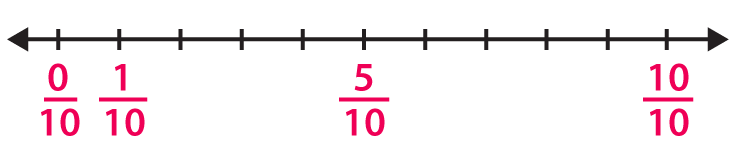3. Represent 2/7, 5/7 and 6/7 on a number line.

Solution:

The fraction 2/7, 5/7 and 6/7 are represented on a number line as given below: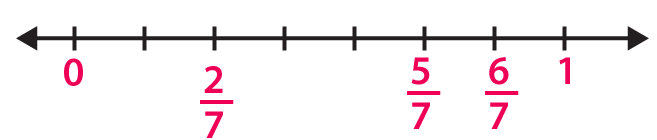4. How many fractions lie between 0 and 1.

Solution:

Infinite number of fractions lie between 0 and 1

This can be done by taking numerator less than denominator in a fraction.

5. Represent 0/8 and 8/8 on a number line.

Solution:

The fraction 0/8 and 8/8 are represented on a number line as given below: